Courses

# Test: Previous Year Questions - Electrochemistry (Level 1)

## 21 Questions MCQ Test Chemistry Class 12 | Test: Previous Year Questions - Electrochemistry (Level 1)

Description
This mock test of Test: Previous Year Questions - Electrochemistry (Level 1) for Class 12 helps you for every Class 12 entrance exam. This contains 21 Multiple Choice Questions for Class 12 Test: Previous Year Questions - Electrochemistry (Level 1) (mcq) to study with solutions a complete question bank. The solved questions answers in this Test: Previous Year Questions - Electrochemistry (Level 1) quiz give you a good mix of easy questions and tough questions. Class 12 students definitely take this Test: Previous Year Questions - Electrochemistry (Level 1) exercise for a better result in the exam. You can find other Test: Previous Year Questions - Electrochemistry (Level 1) extra questions, long questions & short questions for Class 12 on EduRev as well by searching above.
QUESTION: 1

Solution:
QUESTION: 2

### Which of the following reactions is possible at anode ?            [AIEEE-2002]

Solution:
*Multiple options can be correct
QUESTION: 3

### For a cell given below Ag | Ag+ || Cu2+ | Cu  —              +  Ag+ + e- → Ag,      Eº = x  Cu2+ +2e- → Cu,    Eº = y  Eº cell is –                     [AIEEE-2002]

Solution:
*Multiple options can be correct
QUESTION: 4

For a cell reaction involving a two-electron change the standard e.m.f. of the cell is found to be 0.295 V at 25ºC. The equilibrium constant of the reaction at 25º C will be –

[AIEEE-2003]

Solution:
*Multiple options can be correct
QUESTION: 5

Standard reduction electrode potentials of three metals A, B and C are respectively +0.5 V, – 3.0V and – 1.2 V. The reducing powers of these metals are -

[AIEEE-2003]

Solution:
*Multiple options can be correct
QUESTION: 6

For the redox reaction :

Zn (s) + Cu2+ (0.1M) → Zn2+ (1M) + Cu(s) taking place in a cell, Eºcell is 1.10 volt. Ecell for the cell will be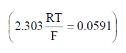[AIEEE-2003]

Solution:
QUESTION: 7

When during electrolysis of a solution of AgNO3 9650 coulombs of charge pass through the electroplating bath, the mass of silver deposited on the cathode will be –

[AIEEE-2003]

Solution:
*Multiple options can be correct
QUESTION: 8

Consider the following E° values

[AIEEE-2004]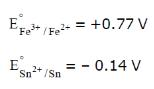under standard conditions the potential for the reaction

Sn(s) + 2Fe3+ (aq) → 2Fe2+ (aq) + Sn2+ (aq) is

Solution:
QUESTION: 9

The standard e.m.f. of a cell, involving one electron change is found to be 0.591 V at 25°C. The equilibrium constant of the reaction is (F = 96500 C mol-1 ; R = 8.314 JK-1mol-1)

[AIEEE-2004]

Solution:
QUESTION: 10

The limiting molar conductivities ^° for NaCl, KBr and KCl are 126, 152 and 150 S cm2 mol-1 respectively. The ^° for NaBr is –

[AIEEE-2004]

Solution:
QUESTION: 11

In a cell that utilises the reaction

Zn(s) + 2H+ (aq)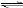Zn2+ (aq) + H2(g)

addition of H2SO4 to cathode compartment, will–

[AIEEE-2004]

Solution:
QUESTION: 12

Aluminium oxide may be electrolysed at 1000ºC to furnish aluminium metal (At. Mass=27 amu ; 1 Faraday = 96,500 Coulombs). The cathode reaction is

Al3+ + 3e-→ Alº

To prepare 5.12 kg of aluminium metal by this method would require -

[AIEEE-2005]

Solution:
QUESTION: 13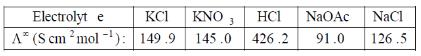Calculate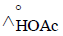using appropriate molar conductance of the electrolytes listed above at infinite dilution in H2O at 25ºC

[AIEEE-2005]

Solution:
QUESTION: 14

For a spontaneous reaction the ΔG, equilibrium constant (K) and Ecell will be respectively -

[AIEEE-2005]

Solution:
QUESTION: 15

Given the data at 25ºC,

Ag + I- → AgI + e-                     Eº = 0.152 V

Ag → Ag+ + e-                          Eº = -0.800 V

What is the value of log Ksp for AgI ?

(2.303 RT/F = 0.059 V)

[AIEEE 2006]

Solution:
QUESTION: 16

The cell, Zn | Zn2+ (1M) | | Cu2+(1M)|Cu (Eºcell)=1.10 V), was allowed to be completely discharged at 298 K.

the relative concentration of zn2+ to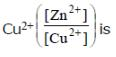Solution:
QUESTION: 17

The equivalent conductance of two strong electrolytes at infinite dilution in H2O (where ions move freely through a solution) at 25ºC are given below -

^°CH3COONa= 91.0 S cm2/equiv

HCl = 426.2 S cm2/equiv

What additional information/quantity one needs to calculate Lº of an aqueous solution of acetic acid ?

[AIEEE 2007]

Solution:
QUESTION: 18

Given EºCr3+/Cr = – 0.72 V, EºFe2+/Fe= – 0.42 V. The potential for the cell Cr |Cr3+ (0.1 M)| |Fe2+ (0.01 M) | Fe is -

[AIEEE 2008]

Solution:
QUESTION: 19

The standard reduction potentials for Zn2+/Zn, Ni2+ /Ni, and Fe2+/Fe are – 0.76, – 0.23 and – 0.44 V respectively. The reaction X + Y2+ → X2+ + Y will be spontaneous when –

[AIEEE 2012]

Solution:
QUESTION: 20

Refining of impure copper with zinc impurity is to be done by electrolysis using electrods as –

[AIEEE-2002]

Solution:
QUESTION: 21

Given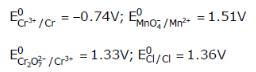Based on the data given above, strongest oxidisting agent will be

[AIEEE 2013]

Solution: